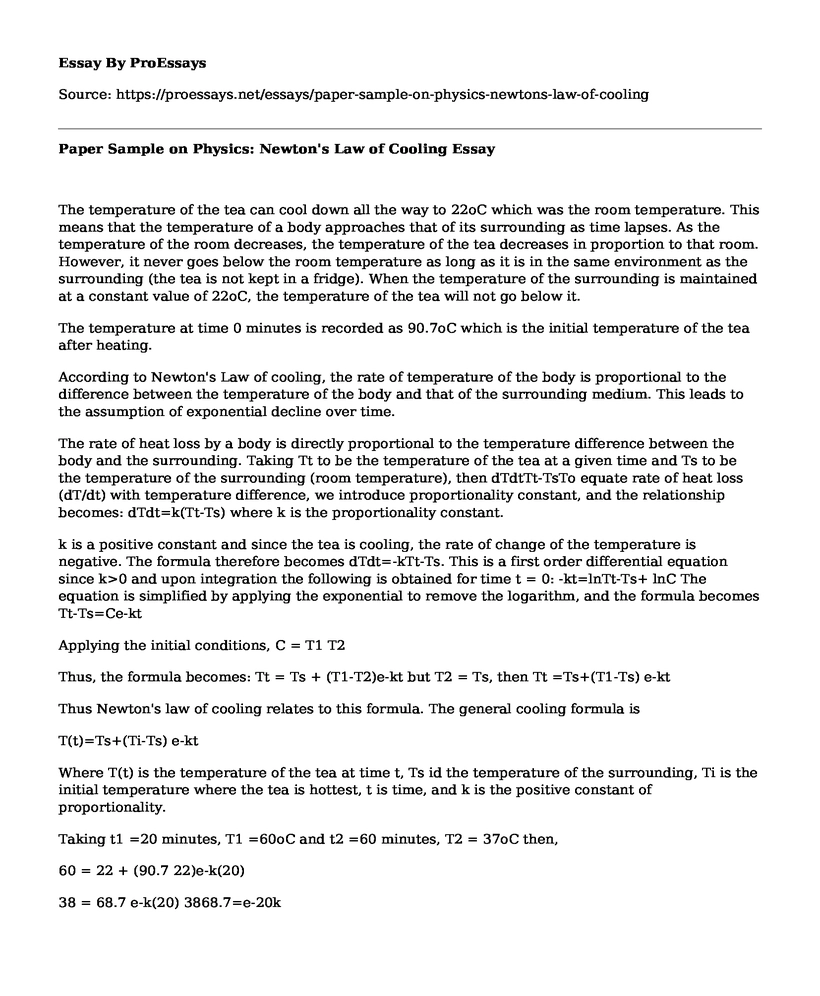# Paper Sample on Physics: Newton's Law of Cooling

 Paper Type:  Problem solving Pages:  2 Wordcount:  503 Words Date:  2021-04-02
Categories:

The temperature of the tea can cool down all the way to 22oC which was the room temperature. This means that the temperature of a body approaches that of its surrounding as time lapses. As the temperature of the room decreases, the temperature of the tea decreases in proportion to that room. However, it never goes below the room temperature as long as it is in the same environment as the surrounding (the tea is not kept in a fridge). When the temperature of the surrounding is maintained at a constant value of 22oC, the temperature of the tea will not go below it.

Is your time best spent reading someone else’s essay? Get a 100% original essay FROM A CERTIFIED WRITER!

The temperature at time 0 minutes is recorded as 90.7oC which is the initial temperature of the tea after heating.

According to Newton's Law of cooling, the rate of temperature of the body is proportional to the difference between the temperature of the body and that of the surrounding medium. This leads to the assumption of exponential decline over time.

The rate of heat loss by a body is directly proportional to the temperature difference between the body and the surrounding. Taking Tt to be the temperature of the tea at a given time and Ts to be the temperature of the surrounding (room temperature), then dTdtTt-TsTo equate rate of heat loss (dT/dt) with temperature difference, we introduce proportionality constant, and the relationship becomes: dTdt=k(Tt-Ts) where k is the proportionality constant.

k is a positive constant and since the tea is cooling, the rate of change of the temperature is negative. The formula therefore becomes dTdt=-kTt-Ts. This is a first order differential equation since k>0 and upon integration the following is obtained for time t = 0: -kt=lnTt-Ts+ lnC The equation is simplified by applying the exponential to remove the logarithm, and the formula becomes Tt-Ts=Ce-kt

Applying the initial conditions, C = T1 T2

Thus, the formula becomes: Tt = Ts + (T1-T2)e-kt but T2 = Ts, then Tt =Ts+(T1-Ts) e-kt

Thus Newton's law of cooling relates to this formula. The general cooling formula is

T(t)=Ts+(Ti-Ts) e-kt

Where T(t) is the temperature of the tea at time t, Ts id the temperature of the surrounding, Ti is the initial temperature where the tea is hottest, t is time, and k is the positive constant of proportionality.

Taking t1 =20 minutes, T1 =60oC and t2 =60 minutes, T2 = 37oC then,

60 = 22 + (90.7 22)e-k(20)

38 = 68.7 e-k(20) 3868.7=e-20k

To find k, we apply the natural logarithm. Thus ln0.55313= -20k k= -0.59216-20=0.02961The value of k is applied to the formula T(t)=Ts+(Ti-Ts) e-kt and the values of T at different times determined. The values are plotted against time to give the cooling curve that is in close relationship to the one obtained during the experiment.

References

Amrita University. (2017). Newton's Law of cooling. Retrieved January 30, 2017, from Value laboratories amrita: http://vlab.amrita.edu/?sub=1&brch=194&sim=354&cnt=1

MathMedics. (2017). Newton's Law of Cooling. Retrieved January 30, 2017, from sosmath: http://www.sosmath.com/diffeq/first/application/newton/newton.html

Paper Sample on Physics: Newton's Law of Cooling. (2021, Apr 02). Retrieved from https://proessays.net/essays/paper-sample-on-physics-newtons-law-of-coolingFree essays can be submitted by anyone,

so we do not vouch for their quality

Want a quality guarantee?
Order from one of our vetted writers instead

If you are the original author of this essay and no longer wish to have it published on the ProEssays website, please click below to request its removal:

Liked this essay sample but need an original one?

Hire a professional with VAST experience!